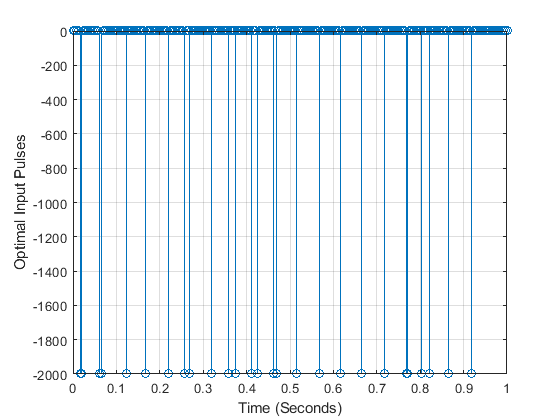# Two Variable Optimization Using Cvx and Gurobi Solver

Dear all,

I have only recently started using CVX optimization tool and I am trying to solve an optimization problem with two variables: x(n) and As, where x(n) is a vector of dimension (nx1) and As is a scalar. A is a matrix of order mxn while b is a vector of order mx1.

In CVX, the problem is described as:

cvx_begin
variable x(n) binary
variable As
minimize(norm(As*A*x - b,2))
subject to
l <= x <= u;
-4000 <= As <=0;
cvx_end


I do understand that if I remove As from the objective function, the problem reduces to a linear constrained integer problem which can be solved using Cvx plus Gurobi solver. However, when I try to optimize both the variables As and x(n) simultaneously, I get the error:

“Disciplined convex programming error:
Only scalar quadratic forms can be specified in CVX”

which again makes sense given the multiplication of the objective function by As and the subsequent two-variable optimization which it represents.

My question is, is there any other way to represent this problem so that it can be solved using CVX?

In particular, As represents the amplitude of the optimization variable x(n) which can vary between 0 and -4000.

1 Like

Reformulate the product of binary and continuous variables per section 2.8 “product values” https://www.fico.com/en/resource-download-file/3217 . You will need to apply this for each variable product, and you will need to make As*A your continuous variables. If you need more help on how to do that, please search on https://or.stackexchange.com/ , and ask a question there if you still need help after doing so.

The resulting program will be an MISOCP (which is a special case of MIDCP), solvable with Gurobi or Mosek as solver.

Alternatively, you can use YALMIP, which has a binmodel command which will do the linearization for you (you will need to bound the variables).

My additional constraint is that variable As should be a constant for all values of x(n), though it can assume any value between -4000 and 0. With your suggestion, the values of As that I will obtain will not be constant but varying for each x(n).

As an example, the optimal input should look something like this:,

where As = -2000. So here x(n) is either 0 or -2000 for all values of variable x.

I suggested how the optimization problem specified in your original post can be implemented in CVX. Now, you apparently want to solve a different optimization problem than you originally stated…

I do not understand your explanation of the problem you now wish to solve. When the optimization problem is solved, each element of x will have one specific value, as will As. So what does “variable As should be a constant for all values of x(n), though it can assume any value between -4000 and 0” mean?

I think you should show what you have implemented. I believe you used different variables where you should have only used one but I am not sure. So basically you want to linearize y = A_s x, which should give

\left\{ \begin{array}{l} -4000x_i \leq y_i \leq 0 \\ -4000(1-x_i) \leq A_s - y_i \leq 0 \end{array} \right. , \ i=1,\dots,n

I believe you have implemented something like

\left\{ \begin{array}{l} -4000x_i \leq y_i \leq 0 \\ -4000(1-x_i) \leq A_{si} - y_i \leq 0 \end{array} \right. , \ i=1,\dots,n

where the variable A_s was duplicated via A_{si}.

(By the way, there is an error in m previous message.)

Apologies. I defined my problem wrongly.

-As is a scalar value which is bounded as follows: -4000<=As<0;
-x(n) is a binary variable which can be either 0 or 1 and belongs to a vector of dimension nx1.

I want to define an optimization problem which gives me two optimal outputs;

1. x vector where x(n) can be 0 or 1.
2. As which is a scalar such that As*x minimizes my cost function.

I hope I explained it clearly now.

Hi Marc. Yes you are right in defining the problem. As is a scalar, while x is a vector of dimension nx1. So, rather than using different variables Asi corresponding to each x(n), I should use only As for all values of x(n) which linearizing y = As*x.

I also implemented your suggestion in the previous comment which you have deleted now but i guess there is an error with the bounds, which makes the problem infeasible. Is that the error you were referring to?

Not at all, I was a little bit too quick with the math and I forgot a term in the objective function which renders the problem not really interesting (don’t bother with it).

And if you use the same variable A_s for all the x_i you should come with a result with the intended behaviour.

Thanks Marc and Mark. Marc, I implemented your suggestion, but from the solution, it seems like there is no relationship between y and x. The new optimization problem that I defined is as follows:

cvx_begin
variable x(n) binary
variable y(n)
variable As
minimize(norm(Ay - b,2))
subject to
l
(1-x(n)) <= As-y(n) <= u*(1-x(n))
cvx_end

where l = -4000 and u = 0. But it seems like the optimization problem is not following the constraints. It is optimizing y irrespective of the constraints of specified.

Do I need to add some extra constraint to explicitly specify the relationship between x, y and As?

Well there is the other constraint in the linearization that was not implemented.

Thanks, here is the updated answer and it works well.

cvx_begin
variable x(n) binary
variable y(n)
variable As
minimize(norm(Ay - b,2))
subject to
l
x <= y <= ux
l
(1-x) <= As-y <= u*(1-x)
cvx_end

In addition, for the constraint definition, I have replaced x(n) and y(n) in the previous solution by x (nx1 vector) and y (nx1 vetor) for the optimization to work properly.